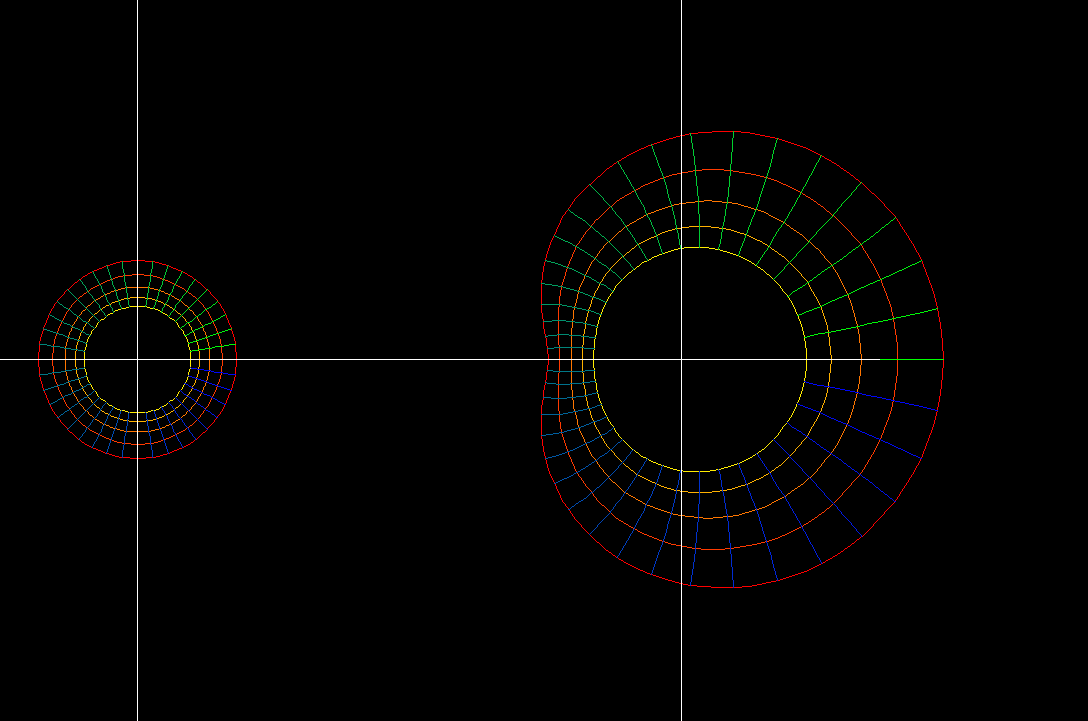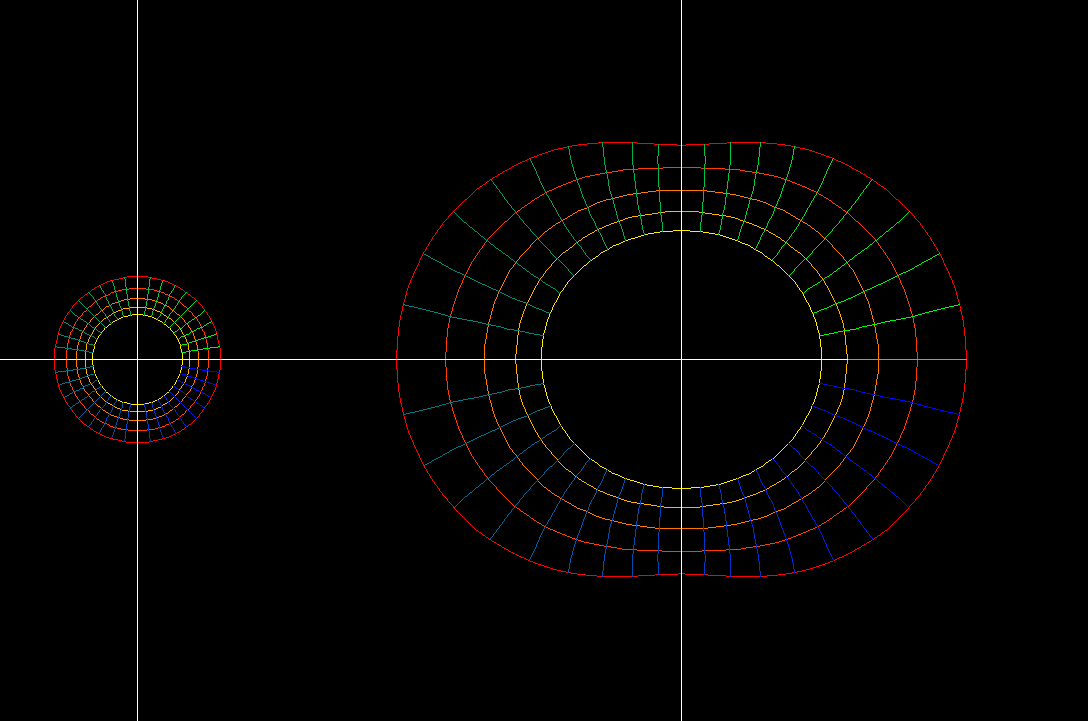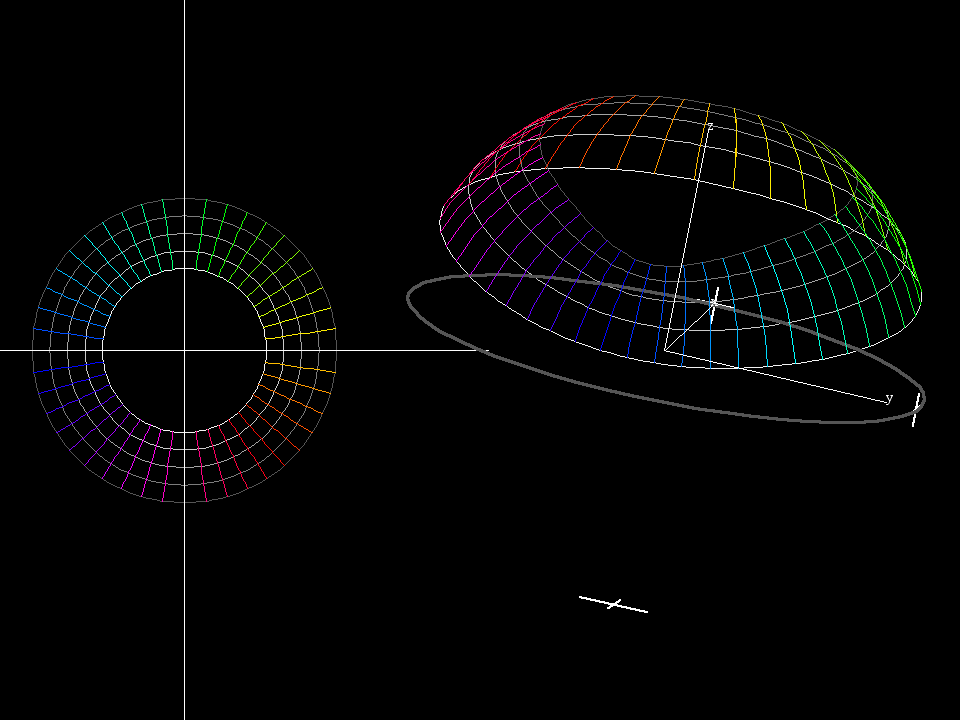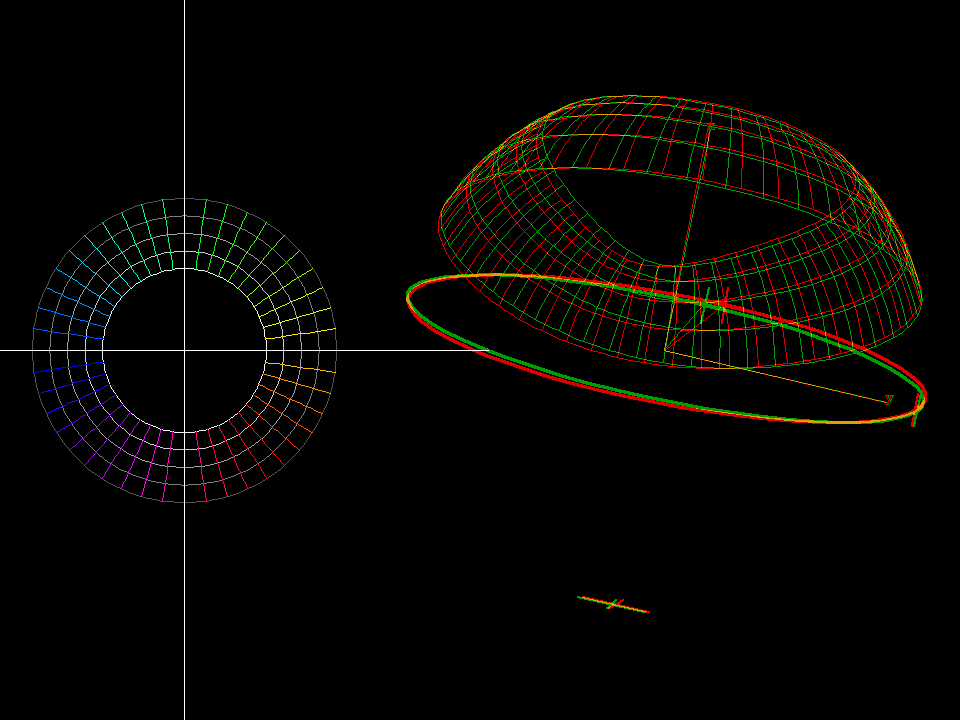# Polynomial Examplemap: z → f(z) = a*z^2 + 2*z domain: 0.7 < |z| < 1.3 morph: 0.5 < a < 1.5 Observe that the slightly curved parameter squares in the domain are mapped to “curved squares” in the range. All angle preserving (=conformal) maps have this property. When a parameter circle of the domain hits the point z0 where f'(z0) = 0 then the image of this circle is a cardioid. The other parameter circles are mapped to limacons.map: z ⟶ f(z) = a*z^3 + 3*z domain: 0.7 ≺ |z| ≺ 1.3 morph: 0.25 ≺ a ≺ 1.25 As for all conformal maps we see: The “curved parameter squares” in the domain are mapped to “curved squares” in the range. Near the zeros of f’ the image squares are very small. The parameter circles which hit the zeros of f' are mapped to Nephroidspower2 riemann 001 The same deformation as before: map: z → f(z) = a*z^2 + 2*z domain: 0.7 < |z| < 1.3 morph: 0.5 < a < 1.5 But the image is shown on the Riemann sphere, using stereographic projection from the Gaussian plane to the Riemann sphere.Same deformation as before on the Riemann sphere. The anaglyph rendering shows the spherical shape of the image much better.• function: `z + c*z^4/4`
• domain: conformal polar grid, `0.8 ≦ |z| ≦ 1.25`, `0 ≦ angle ≦ 2*pi`
• morph : `0.3 ≦ c ≦ 1.0`

Note that the circles `t → exp(i*t)` in the domain are mapped to `exp(i*t) + c*exp(4i*t)` in the range. We recognize them as cycloidal curves. (that is, curve traced by rolling a circle on another circle.)

Compare the inwards pointing cusps of the examples on this page with the outward pointing cusp of A Example of a Non-Conformal Mapping

In this case we have three zeros of the derivative. But the behavior of the map near these critical points is the same as in the earlier cases (near the zeros of their derivative). Observe an important fact of complex analysis: No interior point of the domain is mapped to the boundary of the image. In other words: The boundary of the image set consists only of images of boundary points. No fold lines as in the non-conformal example can occur.

• function: `z + c*z^5/5`
• domain: conformal polar grid, `0.8 ≦ |z| ≦ 1.25`, `0 ≦ angle ≦ 2*pi`
• morph : `0.3 ≦ c ≦ 1.0`